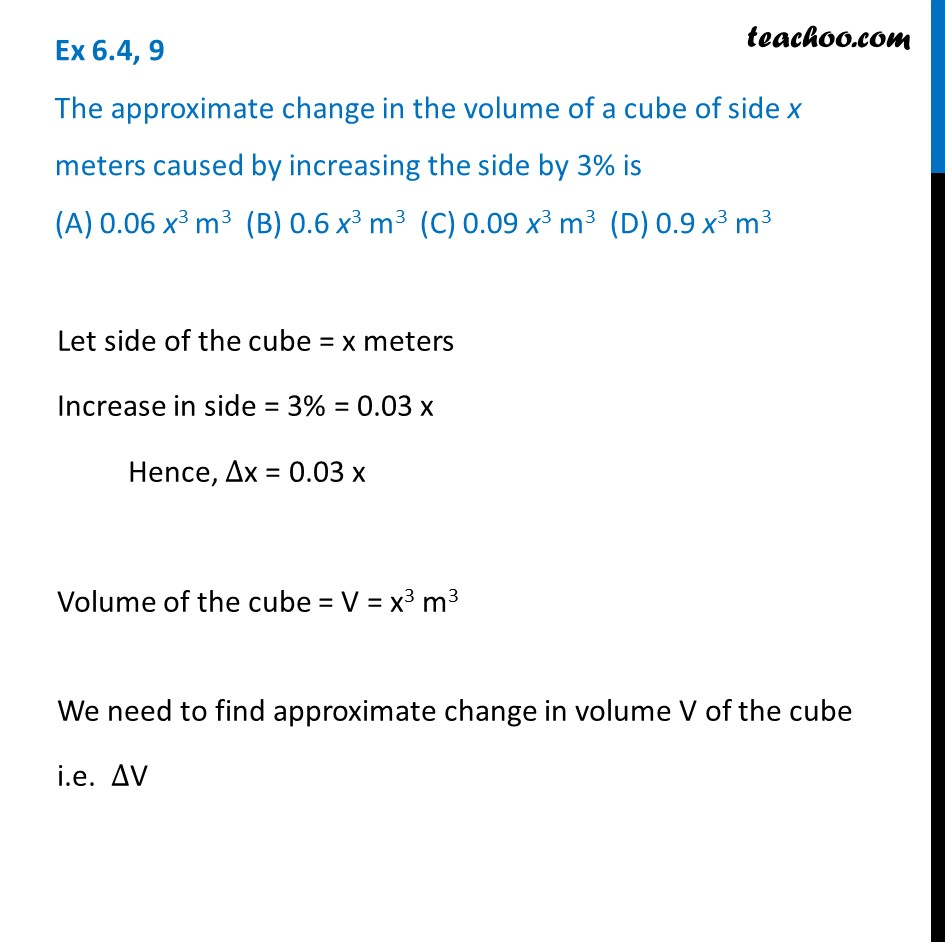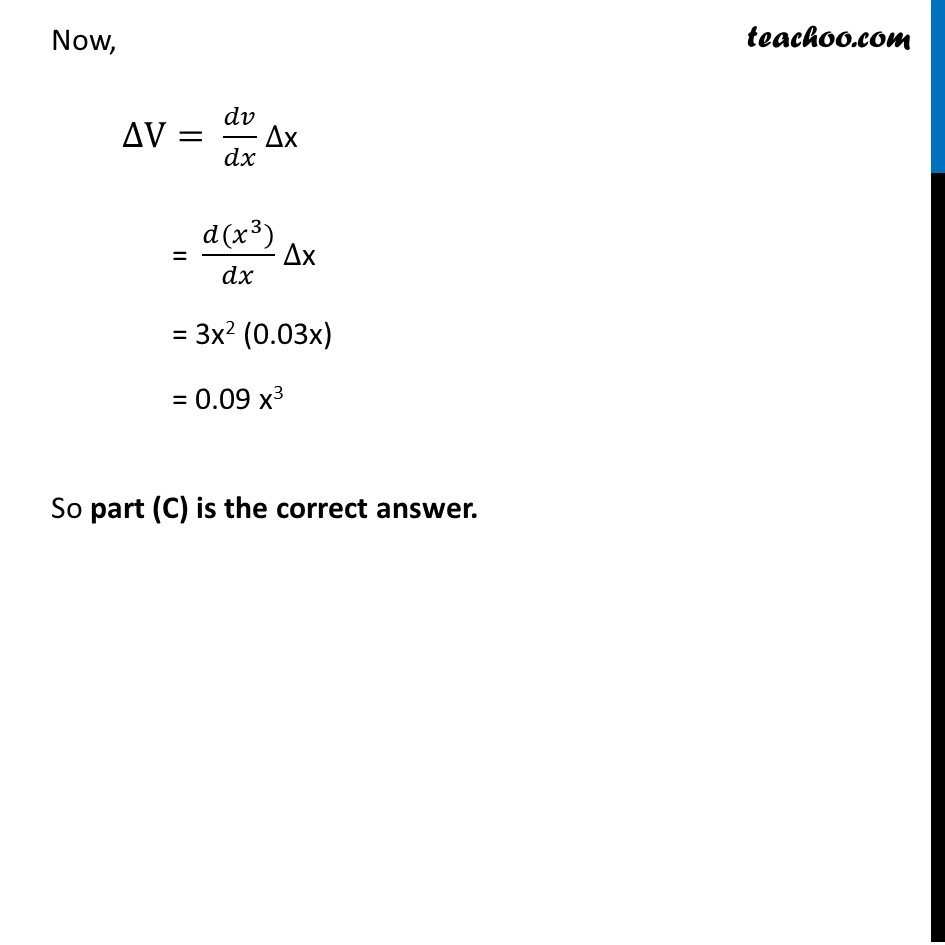Approximations (using Differentiation)

Chapter 6 Class 12 Application of Derivatives
Serial order wiseLearn in your speed, with individual attention - Teachoo Maths 1-on-1 Class

### Transcript

Question 9 The approximate change in the volume of a cube of side x meters caused by increasing the side by 3% is (A) 0.06 x3 m3 (B) 0.6 x3 m3 (C) 0.09 x3 m3 (D) 0.9 x3 m3Let side of the cube = x meters Increase in side = 3% = 0.03 x Hence, ∆x = 0.03 x Volume of the cube = V = x3 m3 We need to find approximate change in volume V of the cube i.e. ∆V Now, ∆V= 𝑑𝑣/𝑑𝑥 ∆x = (𝑑(𝑥^3))/𝑑𝑥 ∆x = 3x2 (0.03x) = 0.09 x3 So part (C) is the correct answer.